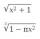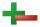# Evaluate expression 2

Evaluate expression with negatives:

(-3)+4+(-8)+(-6)+4+(-1)

x =  -10

### Step-by-step explanation:Did you find an error or inaccuracy? Feel free to write us. Thank you!## Related math problems and questions:

• Evaluate expressionEvaluate expression using BODMAS rule: 1 1/4+1 1/5÷3/5-5/8
• Expression plus minusEvaluate expression: (-1)2 . 12 – 6 : 3 + (-3) . (-2) + 22 – (-3) . 2
• Evaluate - order of opsEvaluate the expression: 32+2[5×(24-6)]-48÷24 Pay attention to the order of operation including integers
• Expression 6Evaluate expression: -6-2(4-8)-9
• Evaluate expressionIf x=2, y=-5 and z=3 what is the value of x-2y
• With bracketCalculate (evaluate) simple mathematical expression with a negative numbers and a bracket: 13+15*5-2*(-6)
• Multiplication negativesJosh says that (4)(-7)(-1) is equal to (4)(7). Evangelina says (4)(-7)(-1) is equal to (-28)(-1) . Who is correct? Explain your reasoning.
• Evaluate 12Evaluate and simplify 9 divided by 7/6.
• Evaluate expressionEvaluate following expression: 5 to the power of 2 - (4x4) divided by 2 =
• Evaluate 9Evaluate expression: 3 { 4 − 4 ( 5 − 12 ) } + 20
• Expression 8Evaluate this expressions: a) 5[3 + 4(2.8 - 3)] b) 5×(8-4)÷4-2
• Evaluate 17Evaluate 2x+6y when x=- 4/5 and y=1/3. Write your answer as a fraction or mixed number in simplest form.
• Evaluate 5Evaluate expression x2−7x+12x−4 when x=−1
• Evaluate expr with 2 varsIf a=6 and x=2, find the value of (2ax + 7x- 10) /(4ax-3a-2)
• Brackets 2Add parenthesis to make true: 5-2×6-4+2=5
• EquatiomSolve equation with negatives: X/(-5) + 2 = -9
• Opposite numbersCalculate opposite numbers (additive inverse) to given ones: